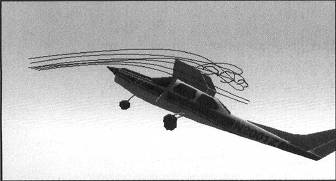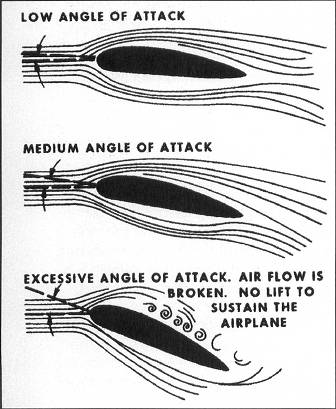# Microsoft Flight Simulator Handbook

by Jonathan M. Stern

Basic Aerodynamics

Theories of Flight

To understand the basics of flight, one must start with a principle discovered by Daniel Bernoulli, an 18th-century Swiss mathematician, Bernoulli discovered that the pressure of a fluid in motion decreases as the rate of movement of the fluid increases. An airfoil, of which a wing is one, is designed to accelerate the flow of air (which is a fluid) over its curved top surface, thereby decreasing the pressure above the wing.

The relatively flat underside of the wing maintains the speed of the air moving beneath it and results in a higher relative pressure on the underside of the wing. The higher pressure under the wing pushes upward on the wing—a force known as lift. A portion of the lift is also created by the force acting equal and opposite to the downward deflection of the air hitting the underside of the wing.

The angle and speed at which the wing moves through the air determines the amount of lift. The necessary lift can be created by an appropriate combination of wing angle and speed. The wing angle, known as angle of attack, is measured by the angle formed between the wing and the relative wind, which is the direction of the airflow in relation to the wing.

At a given weight, the lower the airspeed, the higher the angle of attack needed to maintain level flight. As speed increases, the necessary angle of attack decreases. If the speed decreases, the angle of attack must increase. The angle of attack can be increased to the point that it becomes so great that the air flowing over the upper surface of the wing can no longer follow the wing's curvature. At this point, referred to as the critical angle of attack, a burbling turbulent flow of air causes the airplane to buffet (see Figure 2.10).Figure 2.10. Burbling begins as the airplane reaches its critical angle of attack.

If the critical angle of attack is exceeded, the airplane stalls, a condition that results in insufficient lift to maintain level flight (see Figure 2.11).Figure 2.11. Turbulent flow precedes a full stall.

This occurs in most light airplanes at an angle of attack of between 15 and 20 degrees. One must keep in mind, however, the difference between angle of attack, which is the angle formed between the wing and the flight path, and the angle formed between the wing and the ground. An aerodynamic stall has nothing to do with the condition of the airplane's engine.